Courses

# Ex 9.2 NCERT Solutions- Some Applications of Trigonometry Class 10 Notes | EduRev

## Class 10 : Ex 9.2 NCERT Solutions- Some Applications of Trigonometry Class 10 Notes | EduRev

The document Ex 9.2 NCERT Solutions- Some Applications of Trigonometry Class 10 Notes | EduRev is a part of the Class 10 Course Mathematics (Maths) Class 10.
All you need of Class 10 at this link: Class 10

NCERT TEXTBOOK QUESTIONS SOLVED

EXERCISE 9.2

Q 1. The angles of depression of the top and the bottom of a building 50 m high as observed from the top of a tower are 30° and 60° respectively. Find the height of the tower and also the horizontal distance between the building and the tower.

Sol. In the figure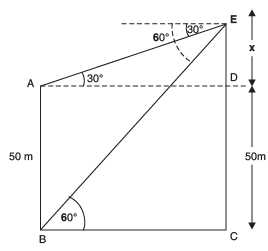Let AB = 50 m be the building.
Let CE be the tower such that CE = (50 + x) m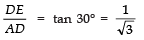⇒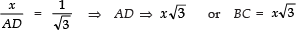...(1)

In right ΔACE, we have: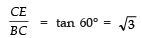⇒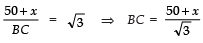...(2)

From (1) and (2), we get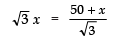⇒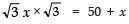⇒ 3x − x =50 ⇒ x = 25

∴ Height of the tower = 50 + x
= 50 + 25
= 75 m

Now from (1), BC = √3 × x
= √3 × 25 m
= 1.732 × 25 m
= 43.25 m

i.e., The horizontal distance between the building and the tower = 43.25 m.

Q 2. The angle of elevation of the top of a tower as observed from a point on the ground is ‘α ’ and on moving ‘a’ metres towards the tower, the angle of elevation is ‘β’. Prove that the height of the tower is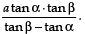Sol. In the figure, let the tower be represented by AB.
∴ In right Δ ABC, we have: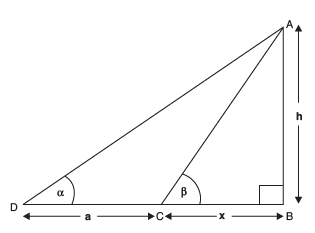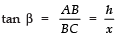⇒ x tan β = h

⇒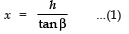Now, in right ΔABD, we have: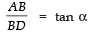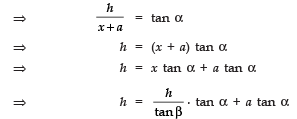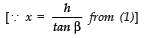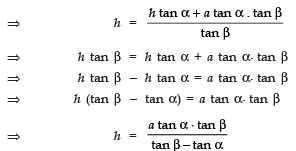Q 3. A vertical tower stands on a horizontal plane and is surmounted by a vertical flag staff of height 5 m. From a point on the plane the angles of elevation of the bottom and top of the flag staff are respectively 30° and 60°. Find the height of the tower.

Sol. Let in the figure, BC be the tower such that

BC = y metres.

CD be the flag staff such that
CD = 5m
⇒ BD =(y + 5) m.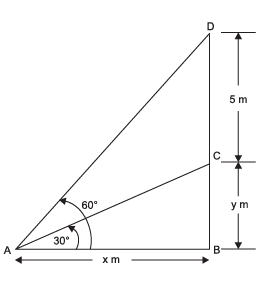In right Δ ABC, we have: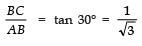⇒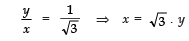...(1)

In right Δ ABD, we have: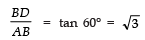⇒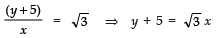∴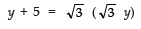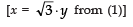⇒ y + 5 = 3 y
⇒ 3y − y = 5 ⇒ y = 5/2  = 2.5 m

∴ The height of the tower = 2.5 m.

Q 4. The length of the shadow of a tower standing on level plane is found to be 20 m longer when the sun’s altitude is 30° than when it was 60°. Find the height of the tower.

Sol. In the figure, let CD be the tower such that
CD = h metres
Also BC = x metres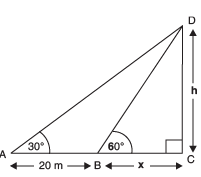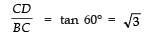⇒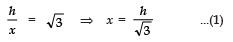In right Δ ACD, we have: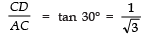⇒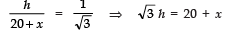⇒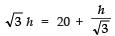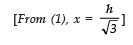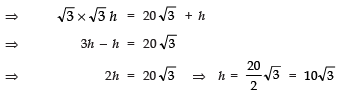Thus, the height of the tower = 17.32 m.

Q 5. From the top of a hill 200 m high, the angles of depression of the top and bottom of a pillar are 30° and 60° respectively. Find the height of the pillar and its distance from the hill.

Sol. In the figure, let AD is the hill such that
AD = 200 m and CE is the pillar.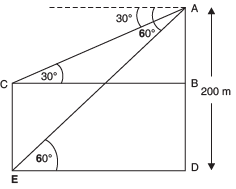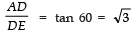∴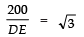⇒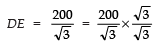⇒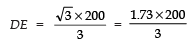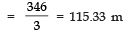⇒ Distance between pillar and hill = 115.33 m

Now,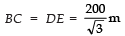[∵ DE = BC]

In right ∆ ABC, we have: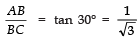⇒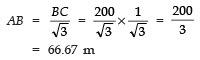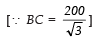∴ Height of the pillar

CE = AD − AB    [∴ CE = BD]
= 200 − 66.67 m
= 133.33 m

Q 6. The angles of elevation of the top of a tower from two points on the ground at distances a and b units from the base of the tower and in the same straight line with it are complementary. Prove that the height of the tower is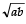units.

Sol. In the figure, AB is the tower, such that: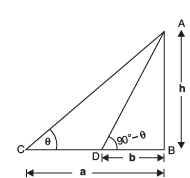AB = h
BD = b
BC = a

In right Δ ABD, we have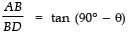⇒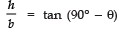⇒  h = b cot θ     ...(1)

In right Δ ABC, we have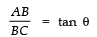⇒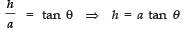..(2)

Multiplying (1) and (2), we get
h × h = b cot θ × a tan θ
⇒ h2 = a × b × (cot θ × tan θ)    [∵ cot θ × tan θ = 1]
⇒ h2 = a b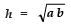Q  7. A pole 5 m high is fixed on the top of a tower. The angle of elevation of the top of the pole observed from a point ‘A’ on the ground is 60° and the angle of depression of the point ‘A’ from the top of the tower is 45°. Find the height of the tower.

Sol. In the figure, let BC be the tower and CD be the pole.
Let BC = x metres and AB = y metres In right ∆ ABC, we get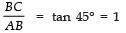⇒ BC = AB ⇒ y = x ... (1)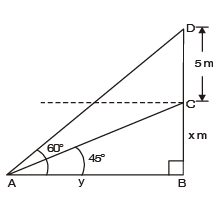In right Δ ABD, we have: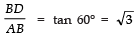⇒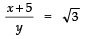⇒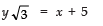⇒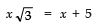∴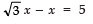[∵ x = y from (1)]
⇒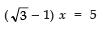⇒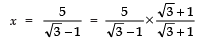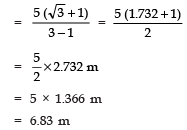Thus, the height of the tower = 6.83 m

Offer running on EduRev: Apply code STAYHOME200 to get INR 200 off on our premium plan EduRev Infinity!

,

,

,

,

,

,

,

,

,

,

,

,

,

,

,

,

,

,

,

,

,

;